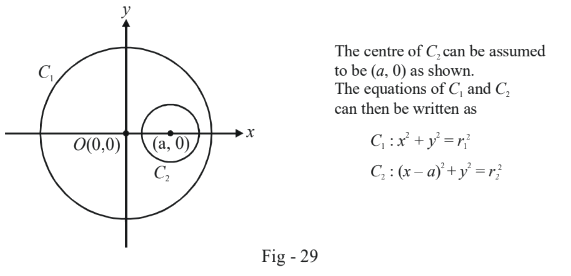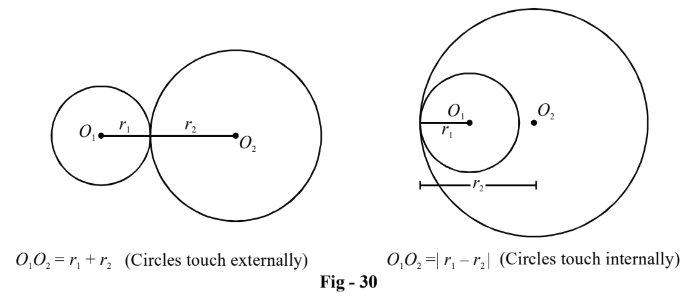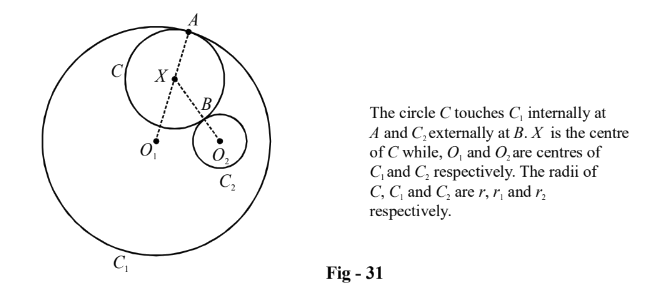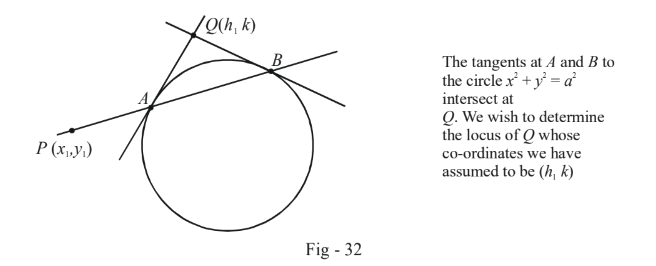# Examples On Tangents To Circles Set-5

Go back to  'Circles'

Example - 29

Let $${C_1}$$ and $${C_2}$$ be two circles with $${C_2}$$ lying inside $${C_1}.$$ A circle $${C}$$ lying inside $${C_1}$$ touches $${C_1}$$ internally and $${C_2}$$ externally. Determine the locus of the centre of $${C}$$.

Solution: First of all, you must note that  is not concentric with $${C_1}.$$  All that is said is that $${C_2}$$  lies somewhere inside $${C_1}.$$

Let us first discuss what all would be involved in solving this question through a co-ordinate approach.

We could first assume a co-ordinate axes, say, with the $$x$$-axis lying along the line joining the centres of $${C_1}$$$${C_1}$$ and $${C_2}$$ and the origin at the centre of $${C_1}:$$We could then assume the equation of $$C$$to be $${x^2} + {y^2} + 2gx + 2fy + c = 0$$and impose the necessary constraints on $$g$$, $$f$$ and $$c$$ so that $$C$$ touches $${C_2}$$ externally and $${C_1}$$ internally. Recall the appropriate constraints for two circles $${S_1}$$ and $${S_2}$$ touching externally and internally.Imposing these constraints gives us the necessary conditions that $$g$$, $$f$$ and $$c$$ must satisfy and hence the locus of the centre of $$C$$ which is $$( - g, - f).$$

However, we will be much letter off in using a pure-geometry approach here also as you’ll soon see.

Assume an arbitrary circle $$C$$ of radius $$r$$ inside $${C_1}$$ with centre $$X$$, and which satisfies the given constraint:By the properties of circles touching internally and externally, we have

\begin{array}{l}\,\,\,\,\,\,\,\,\,\,\,\,\,\,\,\,\,\,&{O_1}X = {O_1}A - AX = {r_1} - r\\\,\,\,\,\,\,\,\,\,\,\,\,\,\,\,\,\,&{O_2}X = {O_2}B + BX = {r_2} + r\\ \Rightarrow \qquad & {O_1}X + {O_2}X = {r_1} + {r_2}\,\,\,\,\,\,\,\,\,\,\,\,\,\,\,\,\,\,\,\,\,\,\,\,\,\,\,\,\,...\left( 1 \right)\end{array}

(1) simply states the centre of $$C$$ i.e. $$x$$, moves in such a way so that the sum of its distances from $${O_1}$$ and $${O_2}$$  is constant. Thus, $$X$$ must lie on an ellipse with $${O_1}$$ and $${O_2}$$  as the two foci !

To sketch the path that $$X$$ can take, we can follow the approach described in the unit on Complex numbers. Fix two pegs at $${O_1}$$ and $${O_2}$$ and tie a string of length $${r_1} + {r_2}$$ between these two pegs. Use your pencil and the taut string as a guide to trace out the ellipse. This is the path on which the centre of $$C$$ can move.

Example - 30

POLE AND POLAR

Through an arbitrary fixed point $$P({x_1},{y_1}),$$ a variable line is drawn intersecting the circle $${x^2} + {y^2} = {a^2}$$ at $$A$$ and $$B$$ respectively. Tangents drawn to this circle at $$A$$ and $$B$$ intersect at $$Q$$. Find the locus of $$Q$$.

Solution:We can view this situation from a different perspective. We have a point $$Q(h,k)$$ from which we draw tangents $$QA$$ and $$QB$$ to the circle $${x^2} + {y^2} = {a^2}.$$ Thus, the equation of $$AB$$ (which is the chord of contact) is

\begin{align}\,\,\,\,\,\,\,\,\,\,\,\,\,\,\,\,\\&T(h,k) = 0 \\ \Rightarrow & hx + ky = {a^2}\end{align}

Now, this chord of contact also passes through  $$P({x_1},{y_1})$$ so that

$h{x_1} + k{y_1} = {a^2}\,\,\,\,\,\,\,\,\,\,\,\,\,\,\,\,\,\,\,\,\,\,\,...(1)$

What we have in (1) is a linear equation involving the variables $$h$$ and $$k$$. Note that and are constant. Thus, we can infer from (1) that $$(h,k)$$ lies on the line

$x{x_1} + y{y_1} = {a^2}\,\,\,\,\,\,\,\,\,\,\,\,\,\,\,\,\,\,\,\,\,\,\,\,\,\,\,\,\,...(2)$

This is the required equation !

The line obtained in (2) is referred to as the polar of the point $$P$$ with respect to the given circle. $$P$$ is itself referred to as the pole of the polar.

Notice that the equation of the polar can be written concisely as

$$\qquad \qquad \fbox{\begin{array}{*{20}{c}} {T({x_1},{y_1}) = 0} \end{array}}$$:Equation of the polar for the pole P$$\left( {{x_1},{y_2}} \right)$$

This example should show you that sometimes enormous simplifications are achieved using a co-ordinate geometrical approach rather than a pure-geometrical one. Co-ordinate geometry is not all that bad!

# TRY YOURSELF - I

Q. 1   Three circle with radii $${r_1},{r_2}$$ and $${r_3}$$ touch each other externally. The tangents at their points of contact meet at a point whose distance from any point of contact is $$4$$. Show geometrically that

$\frac{{{r_1}{r_2}{r_3}}}{{{r_1} + {r_2} + {r_3}}} = 16$

Q. 2   What is the equation of the tangent to $${x^2} + {y^2} - 30x + 6y + 109 = 0$$  at $$({4_1} - 1)?$$

Q. 3   Find the tangents to $${x^2} + {y^2} - 6x + 4y - 12 = 0$$ parallel to $$4x + 3y + 5 = 0.$$

Q. 4   Find the tangents to $${x^2} + {y^2} = {a^2}$$ which make a triangle of area $${a^2}$$ with the axes.

Q. 5   If $$P(a,b)$$ and $$Q(b,a)$$  are two points $$(b \ne a),$$ find the equation of the circle touching $$OP$$ and $$OQ$$ at $$P$$ and $$Q$$ where $$O$$ is the origin .

Q. 6   Find the equation of the normal to $$3{x^2} + 3{y^2} - 4x - 6y = 0$$ at (0, 0)

Q. 7   The line $$2x - y + 1 = 0$$ is a tangent to a circle at $$(2, 5)$$; its centre lies on $$x + y = 9.$$  Find its equation.

Q. 8   If $$3x + y = 0$$ is a tangent to a circle whose centre is $$(2, –1)$$, find the other tangent to the circle from the origin.

Q. 9   Find the locus of the point of intersection of tangents to the circle $${x^2} + {y^2} + 4x - 6x + 9{\sin ^2}\alpha + 13{\cos ^2}\alpha = 0$$ which are  inclined at an angle of $$2\alpha$$ to each other.

Q. 10 Prove that the intercept of the pair of tangents from the origin to $${x^2} + {y^2} + 2gx + 2fy + {k^2} = 0,$$ on the line $$y = \lambda$$ is \begin{align}\frac{{2\lambda k}}{{{k^2} - {g^2}}}\end{align}.